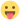## Cohen's d in post-hoc test

Discuss the jamovi platform, possible improvements, etc.

## Cohen's d in post-hoc test

Hi,

I have performed a mixed 2x2 ANOVA and I noticed that the post-hoc test does not report the effect size. Is there any possibility to implement it?

On the other hand, if JAMOVI computes Cohen's d by the formula d = t/sqrt(n), it would be correct to compute Cohen's d by using the t value reported by JAMOVI and the n value of the respective group?

Please, see the attached file for more details

I accept any other suggestion, thanks in advance
AttachmentsPost-hoc test table
Captura de pantalla 2019-10-25 a las 22.12.43.png (115.35 KiB) Viewed 2595 times
Vicente_inefo

Posts: 4
Joined: Wed May 29, 2019 11:39 pm

## Re: Cohen's d in post-hoc test

hi,

the issue is that the post-hoc tests in jamovi are based on the estimated marginal means, rather than simply being pairwise comparisons of the raw data. so calculating cohen's d for these is a bit more complicated ... i *think* it's possible to calculate cohen's d for emmeans, but even then i'm not completely sure.

i've asked a few people about it, and i'll see what they come back with.

cheersjonathon

Posts: 1282
Joined: Fri Jan 27, 2017 10:04 am

## Re: Cohen's d in post-hoc test

Thank you very much, I will remain attentive to your response
Vicente_inefo

Posts: 4
Joined: Wed May 29, 2019 11:39 pm

## Re: Cohen's d in post-hoc test

Hi,

I was also confused to find that the Cohen's d calculated with the post-hoc comparisons does not match the classical (y1-y2)/S formula. It was useful to learn from Jonathon that the values are based on estimated marginal means. However, I'd still be interested in Cohen's d based on the observed means for teaching purposes, so I'm wondering if anyone can help me find an option to obtain these with jamovi?

Cheers,
José
jose.lopez

Posts: 1
Joined: Mon Feb 10, 2020 11:52 am

## Re: Cohen's d in post-hoc test

hey,

yeah, we'd like to provide a 'pairwise' comparisons module, that works on descriptives, rather than emms. don't get me wrong, i think working off descriptives is wrong most of the timebut it seems to be something a lot of people want.

cheers

jonathonjonathon

Posts: 1282
Joined: Fri Jan 27, 2017 10:04 am

## Re: Cohen's d in post-hoc test

It may be that people are skeptical of emmeans because they're not sure exactly how the estimated marginals relate to the regular old marginals.

I think in the case of an ANOVA, the estimated marginal means are identical to the marginal means UNLESS you're averaging over the levels of a know factor such that the averaged-over levels have unequal sample sizes. In the latter case, the estimated marginal mean are the means of the averaged-over means, rather than the means of raw data. Thus for example, if there are scores for left- and right-handed men and women, and if there are 10 left-handed and 90 right-handed participants, and if the ANOVA model includes handedness and gender as factors, and if one is interested in looking just at the main effect of gender then:

The estimated marginal means for the main effect of gender are not the mean scores for the women versus the mean scores for the men. Rather they are the mean of two means--the mean score for left-handed women and the mean score for right-handed women--versus the mean of two other means--the mean score for left-handed men and the mean score for right-handed men. Thus the estimated marginal means are the means that one would find had the study included an equal number of left- and right-handed participants.

But I don't know how to calculate the variances that correspond to those estimated marginal means (when the sizes of the averaged samples are unequal). This is because it's more complicated: It involves not just pooling variances (consistent with the equal-variance assumption) but also modeling what the variances would be had the sample sizes for the averaged categories been equal.

(Of course, all of this is even more complicated when the dealing with ANCOVA.)
reason180

Posts: 46
Joined: Mon Jul 24, 2017 4:56 pm

## Re: Cohen's d in post-hoc test

The easiest way is to use the t-test. d=t*sqrt(1/n1+1/n2)mcfanda@gmail.com

Posts: 195
Joined: Thu Mar 23, 2017 9:24 pm

## Re: Cohen's d in post-hoc test

mcfanda@gmail.com wrote:The easiest way is to use the t-test. d=t*sqrt(1/n1+1/n2)

I was talking about a within subject effect size (i.e. pre-test vs post-test). I think that JAMOVI computes Cohen's d of the paired samples t-test using the formula d = t/sqrt(n).

In this case, it would be correct to compute Cohen's d by using the t value reported by JAMOVI and the n value of the respective pairs of groups? O maybe, simply use the Cohen's d computed in a paired t-test executed in a different analysis?

Vicente_inefo

Posts: 4
Joined: Wed May 29, 2019 11:39 pm

## Re: Cohen's d in post-hoc test

yup. if you want to calculate a cohen's d for the mean of the difference between two measurements (i.e. a paired samples design), then that's a paired-samples t-test model, and you should use the cohen's d estimated in the paired samples t-test.

the cohen's d we report, is always appropriate for the model you are fitting.

if you want a different cohen's d, you want a different model.

jonathonjonathon

Posts: 1282
Joined: Fri Jan 27, 2017 10:04 am